17 Sound

17.7 The Doppler Effect

Learning Objectives

By the end of this section, you will be able to:

• Explain the change in observed frequency as a moving source of sound approaches or departs from a stationary observer
• Explain the change in observed frequency as an observer moves toward or away from a stationary source of sound

The characteristic sound of a motorcycle buzzing by is an example of the Doppler effect. Specifically, if you are standing on a street corner and observe an ambulance with a siren sounding passing at a constant speed, you notice two characteristic changes in the sound of the siren. First, the sound increases in loudness as the ambulance approaches and decreases in loudness as it moves away, which is expected. But in addition, the high-pitched siren shifts dramatically to a lower-pitched sound. As the ambulance passes, the frequency of the sound heard by a stationary observer changes from a constant high frequency to a constant lower frequency, even though the siren is producing a constant source frequency. The closer the ambulance brushes by, the more abrupt the shift. Also, the faster the ambulance moves, the greater the shift. We also hear this characteristic shift in frequency for passing cars, airplanes, and trains.

The Doppler effect is an alteration in the observed frequency of a sound due to motion of either the source or the observer. Although less familiar, this effect is easily noticed for a stationary source and moving observer. For example, if you ride a train past a stationary warning horn, you will hear the horn’s frequency shift from high to low as you pass by. The actual change in frequency due to relative motion of source and observer is called a Doppler shift. The Doppler effect and Doppler shift are named for the Austrian physicist and mathematician Christian Johann Doppler (1803–1853), who did experiments with both moving sources and moving observers. Doppler, for example, had musicians play on a moving open train car and also play standing next to the train tracks as a train passed by. Their music was observed both on and off the train, and changes in frequency were measured.

What causes the Doppler shift? (Figure) illustrates sound waves emitted by stationary and moving sources in a stationary air mass. Each disturbance spreads out spherically from the point at which the sound is emitted. If the source is stationary, then all of the spheres representing the air compressions in the sound wave are centered on the same point, and the stationary observers on either side hear the same wavelength and frequency as emitted by the source (case a). If the source is moving, the situation is different. Each compression of the air moves out in a sphere from the point at which it was emitted, but the point of emission moves. This moving emission point causes the air compressions to be closer together on one side and farther apart on the other. Thus, the wavelength is shorter in the direction the source is moving (on the right in case b), and longer in the opposite direction (on the left in case b). Finally, if the observers move, as in case (c), the frequency at which they receive the compressions changes. The observer moving toward the source receives them at a higher frequency, and the person moving away from the source receives them at a lower frequency.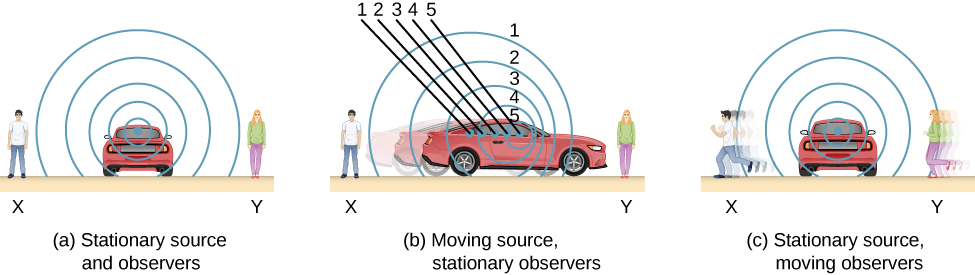Figure 17.30 Sounds emitted by a source spread out in spherical waves. (a) When the source, observers, and air are stationary, the wavelength and frequency are the same in all directions and to all observers. (b) Sounds emitted by a source moving to the right spread out from the points at which they were emitted. The wavelength is reduced, and consequently, the frequency is increased in the direction of motion, so that the observer on the right hears a higher-pitched sound. The opposite is true for the observer on the left, where the wavelength is increased and the frequency is reduced. (c) The same effect is produced when the observers move relative to the source. Motion toward the source increases frequency as the observer on the right passes through more wave crests than she would if stationary. Motion away from the source decreases frequency as the observer on the left passes through fewer wave crests than he would if stationary.

We know that wavelength and frequency are related by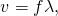where v is the fixed speed of sound. The sound moves in a medium and has the same speed v in that medium whether the source is moving or not. Thus, f multiplied by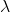is a constant. Because the observer on the right in case (b) receives a shorter wavelength, the frequency she receives must be higher. Similarly, the observer on the left receives a longer wavelength, and hence he hears a lower frequency. The same thing happens in case (c). A higher frequency is received by the observer moving toward the source, and a lower frequency is received by an observer moving away from the source. In general, then, relative motion of source and observer toward one another increases the received frequency. Relative motion apart decreases frequency. The greater the relative speed, the greater the effect.

The Doppler effect occurs not only for sound, but for any wave when there is relative motion between the observer and the source. Doppler shifts occur in the frequency of sound, light, and water waves, for example. Doppler shifts can be used to determine velocity, such as when ultrasound is reflected from blood in a medical diagnostic. The relative velocities of stars and galaxies is determined by the shift in the frequencies of light received from them and has implied much about the origins of the universe. Modern physics has been profoundly affected by observations of Doppler shifts.

Derivation of the Observed Frequency due to the Doppler Shift

Consider two stationary observers X and Y in (Figure), located on either side of a stationary source. Each observer hears the same frequency, and that frequency is the frequency produced by the stationary source.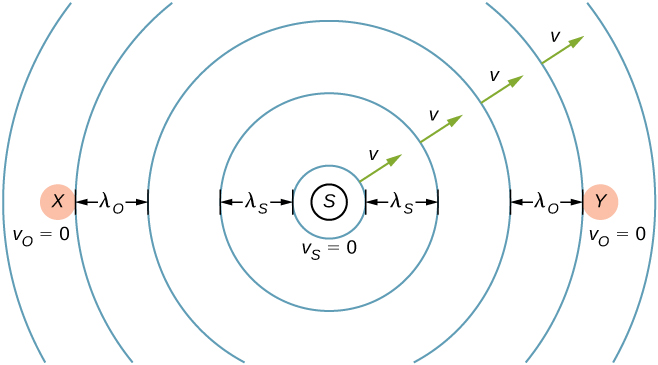Figure 17.31 A stationary source sends out sound waves at a constant frequency     with a constant wavelength     at the speed of sound v. Two stationary observers X and Y, on either side of the source, observe a frequency     , with a wavelength

Now consider a stationary observer X with a source moving away from the observer with a constant speed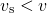((Figure)). At time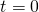, the source sends out a sound wave, indicated in black. This wave moves out at the speed of sound v. The position of the sound wave at each time interval of period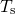is shown as dotted lines. After one period, the source has moved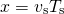and emits a second sound wave, which moves out at the speed of sound. The source continues to move and produce sound waves, as indicated by the circles numbered 3 and 4. Notice that as the waves move out, they remained centered at their respective point of origin.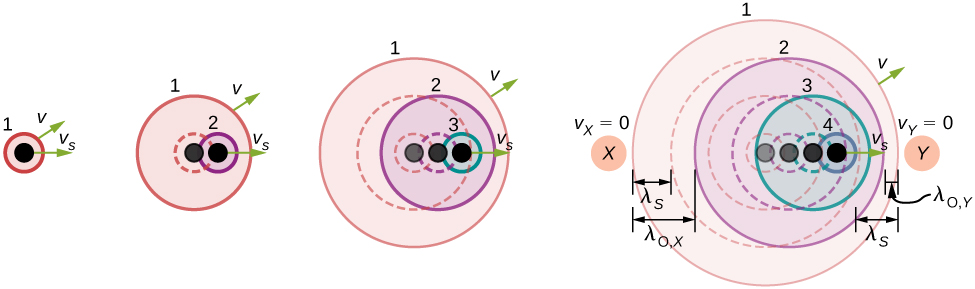Figure 17.32 A source moving at a constant speed     away from an observer X. The moving source sends out sound waves at a constant frequency     with a constant wavelength     , at the speed of sound v. Snapshots of the source at an interval of     are shown as the source moves away from the stationary observer X. The solid lines represent the position of the sound waves after four periods from the initial time. The dotted lines are used to show the positions of the waves at each time period. The observer hears a wavelength of     .

Using the fact that the wavelength is equal to the speed times the period, and the period is the inverse of the frequency, we can derive the observed frequency: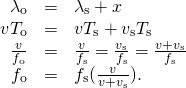As the source moves away from the observer, the observed frequency is lower than the source frequency.

Now consider a source moving at a constant velocity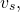moving toward a stationary observer Y, also shown in (Figure). The wavelength is observed by Y as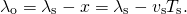Once again, using the fact that the wavelength is equal to the speed times the period, and the period is the inverse of the frequency, we can derive the observed frequency: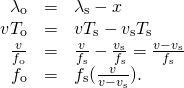When a source is moving and the observer is stationary, the observed frequency is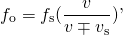where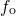is the frequency observed by the stationary observer,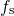is the frequency produced by the moving source, v is the speed of sound,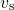is the constant speed of the source, and the top sign is for the source approaching the observer and the bottom sign is for the source departing from the observer.
What happens if the observer is moving and the source is stationary? If the observer moves toward the stationary source, the observed frequency is higher than the source frequency. If the observer is moving away from the stationary source, the observed frequency is lower than the source frequency. Consider observer X in (Figure) as the observer moves toward a stationary source with a speed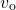. The source emits a tone with a constant frequencyand constant period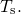The observer hears the first wave emitted by the source. If the observer were stationary, the time for one wavelength of sound to pass should be equal to the period of the sourceSince the observer is moving toward the source, the time for one wavelength to pass is less thanand is equal to the observed period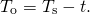At time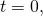the observer starts at the beginning of a wavelength and moves toward the second wavelength as the wavelength moves out from the source. The wavelength is equal to the distance the observer traveled plus the distance the sound wave traveled until it is met by the observer: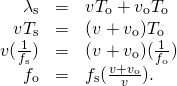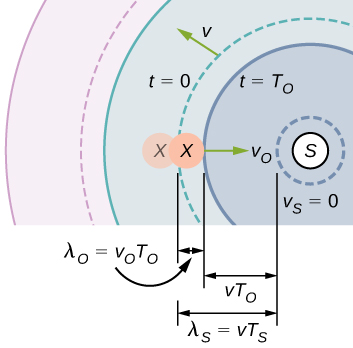Figure 17.33 A stationary source emits a sound wave with a constant frequency     , with a constant wavelength     moving at the speed of sound v. Observer X moves toward the source with a constant speed     , and the figure shows the initial and final position of observer X. Observer X observes a frequency higher than the source frequency. The solid lines show the position of the waves at     . The dotted lines show the position of the waves at     .

If the observer is moving away from the source ((Figure)), the observed frequency can be found: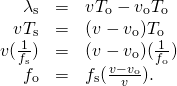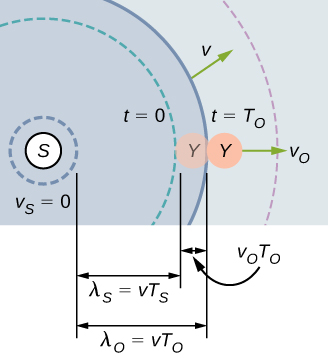Figure 17.34 A stationary source emits a sound wave with a constant frequency     , with a constant wavelength     moving at the speed of sound v. Observer Y moves away from the source with a constant speed     , and the figure shows initial and final position of the observer Y. Observer Y observes a frequency lower than the source frequency. The solid lines show the position of the waves at     . The dotted lines show the position of the waves at     .

The equations for an observer moving toward or away from a stationary source can be combined into one equation: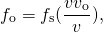whereis the observed frequency,is the source frequency,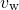is the speed of sound,is the speed of the observer, the top sign is for the observer approaching the source and the bottom sign is for the observer departing from the source.

(Figure) and (Figure) can be summarized in one equation (the top sign is for approaching) and is further illustrated in (Figure):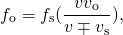Doppler shift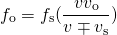Stationary observer Observer moving towards source Observer moving away from source
Stationary source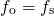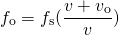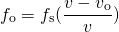Source moving towards observer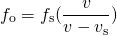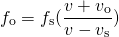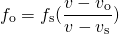Source moving away from observer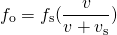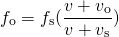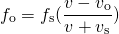where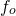is the observed frequency,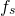is the source frequency,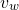is the speed of sound,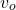is the speed of the observer,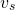is the speed of the source, the top sign is for approaching and the bottom sign is for departing.

The Doppler effect involves motion and a video will help visualize the effects of a moving observer or source. This video shows a moving source and a stationary observer, and a moving observer and a stationary source. It also discusses the Doppler effect and its application to light.

Example

Calculating a Doppler Shift

Suppose a train that has a 150-Hz horn is moving at 35.0 m/s in still air on a day when the speed of sound is 340 m/s.

(a) What frequencies are observed by a stationary person at the side of the tracks as the train approaches and after it passes?

(b) What frequency is observed by the train’s engineer traveling on the train?

Strategy

To find the observed frequency in (a), we must use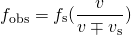because the source is moving. The minus sign is used for the approaching train, and the plus sign for the receding train. In (b), there are two Doppler shifts—one for a moving source and the other for a moving observer.

Solution

1. Enter known values into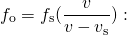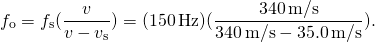Calculate the frequency observed by a stationary person as the train approaches: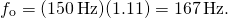Use the same equation with the plus sign to find the frequency heard by a stationary person as the train recedes: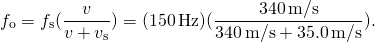Calculate the second frequency: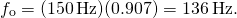2. Identify knowns:
• It seems reasonable that the engineer would receive the same frequency as emitted by the horn, because the relative velocity between them is zero.
• Relative to the medium (air), the speeds are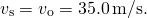• The first Doppler shift is for the moving observer; the second is for the moving source.

Use the following equation: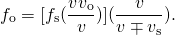The quantity in the square brackets is the Doppler-shifted frequency due to a moving observer. The factor on the right is the effect of the moving source.

Because the train engineer is moving in the direction toward the horn, we must use the plus sign for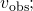however, because the horn is also moving in the direction away from the engineer, we also use the plus sign for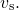But the train is carrying both the engineer and the horn at the same velocity, so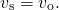As a result, everything butcancels, yielding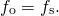Significance

For the case where the source and the observer are not moving together, the numbers calculated are valid when the source (in this case, the train) is far enough away that the motion is nearly along the line joining source and observer. In both cases, the shift is significant and easily noticed. Note that the shift is 17.0 Hz for motion toward and 14.0 Hz for motion away. The shifts are not symmetric.

For the engineer riding in the train, we may expect that there is no change in frequency because the source and observer move together. This matches your experience. For example, there is no Doppler shift in the frequency of conversations between driver and passenger on a motorcycle. People talking when a wind moves the air between them also observe no Doppler shift in their conversation. The crucial point is that source and observer are not moving relative to each other.

Describe a situation in your life when you might rely on the Doppler shift to help you either while driving a car or walking near traffic.

If I am driving and I hear Doppler shift in an ambulance siren, I would be able to tell when it was getting closer and also if it has passed by. This would help me to know whether I needed to pull over and let the ambulance through.

The Doppler effect and the Doppler shift have many important applications in science and engineering. For example, the Doppler shift in ultrasound can be used to measure blood velocity, and police use the Doppler shift in radar (a microwave) to measure car velocities. In meteorology, the Doppler shift is used to track the motion of storm clouds; such “Doppler Radar” can give the velocity and direction of rain or snow in weather fronts. In astronomy, we can examine the light emitted from distant galaxies and determine their speed relative to ours. As galaxies move away from us, their light is shifted to a lower frequency, and so to a longer wavelength—the so-called red shift. Such information from galaxies far, far away has allowed us to estimate the age of the universe (from the Big Bang) as about 14 billion years.

Summary

• The Doppler effect is an alteration in the observed frequency of a sound due to motion of either the source or the observer.
• The actual change in frequency is called the Doppler shift.

Conceptual Questions

Is the Doppler shift real or just a sensory illusion?

Three stationary observers observe the Doppler shift from a source moving at a constant velocity. The observers are stationed as shown below. Which observer will observe the highest frequency? Which observer will observe the lowest frequency? What can be said about the frequency observed by observer 3?[hidden-answer a=”237781″]Observer 1 will observe the highest frequency. Observer 2 will observe the lowest frequency. Observer 3 will hear a higher frequency than the source frequency, but lower than the frequency observed by observer 1, as the source approaches and a lower frequency than the source frequency, but higher than the frequency observed by observer 1, as the source moves away from observer 3.[/hidden-answer]

Shown below is a stationary source and moving observers. Describe the frequencies observed by the observers for this configuration.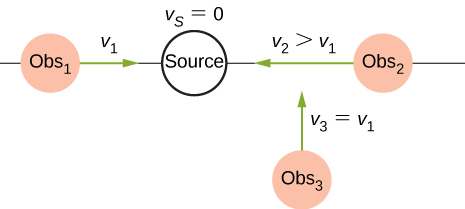Prior to 1980, conventional radar was used by weather forecasters. In the 1960s, weather forecasters began to experiment with Doppler radar. What do you think is the advantage of using Doppler radar?

Doppler radar can not only detect the distance to a storm, but also the speed and direction at which the storm is traveling.

Problems

(a) What frequency is received by a person watching an oncoming ambulance moving at 110 km/h and emitting a steady 800-Hz sound from its siren? The speed of sound on this day is 345 m/s. (b) What frequency does she receive after the ambulance has passed?

a. 878 Hz; b. 735 Hz

(a) At an air show a jet flies directly toward the stands at a speed of 1200 km/h, emitting a frequency of 3500 Hz, on a day when the speed of sound is 342 m/s. What frequency is received by the observers? (b) What frequency do they receive as the plane flies directly away from them?

What frequency is received by a mouse just before being dispatched by a hawk flying at it at 25.0 m/s and emitting a screech of frequency 3500 Hz? Take the speed of sound to be 331 m/s.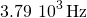A spectator at a parade receives an 888-Hz tone from an oncoming trumpeter who is playing an 880-Hz note. At what speed is the musician approaching if the speed of sound is 338 m/s?

A commuter train blows its 200-Hz horn as it approaches a crossing. The speed of sound is 335 m/s. (a) An observer waiting at the crossing receives a frequency of 208 Hz. What is the speed of the train? (b) What frequency does the observer receive as the train moves away?

a. 12.9 m/s; b. 193 Hz

Can you perceive the shift in frequency produced when you pull a tuning fork toward you at 10.0 m/s on a day when the speed of sound is 344 m/s? To answer this question, calculate the factor by which the frequency shifts and see if it is greater than 0.300%.

Two eagles fly directly toward one another, the first at 15.0 m/s and the second at 20.0 m/s. Both screech, the first one emitting a frequency of 3200 Hz and the second one emitting a frequency of 3800 Hz. What frequencies do they receive if the speed of sound is 330 m/s?

The first eagle hears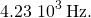The second eagle hears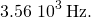Student A runs down the hallway of the school at a speed of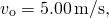carrying a ringing 1024.00-Hz tuning fork toward a concrete wall. The speed of sound is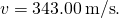Student B stands at rest at the wall. (a) What is the frequency heard by student B? (b) What is the beat frequency heard by student A?

An ambulance with a siren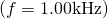blaring is approaching an accident scene. The ambulance is moving at 70.00 mph. A nurse is approaching the scene from the opposite direction, running at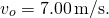What frequency does the nurse observe? Assume the speed of sound is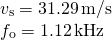The frequency of the siren of an ambulance is 900 Hz and is approaching you. You are standing on a corner and observe a frequency of 960 Hz. What is the speed of the ambulance (in mph) if the speed of sound is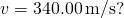What is the minimum speed at which a source must travel toward you for you to be able to hear that its frequency is Doppler shifted? That is, what speed produces a shift of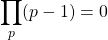on a day when the speed of sound is 331 m/s?

An audible shift occurs when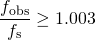;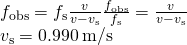Glossary

Doppler effect
alteration in the observed frequency of a sound due to motion of either the source or the observer
Doppler shift
actual change in frequency due to relative motion of source and observer